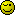# ++variable vs variable++

Hi all,

I’m reading Sitepoint’s Simply JavaScript at the moment and am a bit confused by some information on page 26.

Here, Cameron Adams is trying to explain the difference between:

var age=26;
var ageCopy = age++;

and

var age=26;
var ageCopy = ++age;

He says that in the first example, ageCopy is equal to 26, but in the second example, ageCopy == 27.

I don’t understand this - isn’t ageCopy equal to 27 in both of these examples? What is the actual difference between these statements?

No

ageCopy = age++ - increments and then assigns

ageCopy = ++age - assigns and then increments

In both cases age ends up as 27 but not ageCopy

ORLY? Are you sure it isn’t the other way around?Sorry got that completely the wrong way around

ageCopy = age++ - assigns and then increments

ageCopy = ++age - increments and then assigns

Example:

``````
var a = 1;

var a = 1;
``````

++var is only ever needed in some very special cases. var++ will suffice for 99,99% of the cases where you need to increment a variableThe Major difference between ++a , a++ is the manner of increment and assignment.

in first case agecopy=age++
let the value of age is 26
in that case agecopy first assigns the non incremented value if age.
later compiler increments the age value to 1 and the final age value
becomes 27

in second case agecopy=++age
let the value of age is 26
now compiler increment the value of age to 1 and final age value becomes
27.
later it compiler assigns the incremented value to agevalue.
and ultimately agevalue becomes 27.

I had the same problem the first time I read that in Simply Javascript.

I think it’s a badly-done example. If he had added some more code afterwards, it would have been clearer.

Yeah, I agree with you - it could do with a few extra lines of explanation.

Thanks for the help everyone, I understand it now.